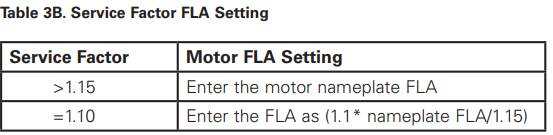# How to determine over load trip setting for C440 relay from motor FLA rating ?

To calculate the over load trip setting, it is not only the FLA which is considered, we also need to consider the service factor, there are two cases with which the FLA is calculated :if the SF is >1.15 then the motor FLA is used as a setting and if SF <= 1.10 then the formula stated in the table above is used.

example :

SF = 1.00

FLA = 89.00 A

then the setting should be : 1.0*89.0/1.15 = 77.4